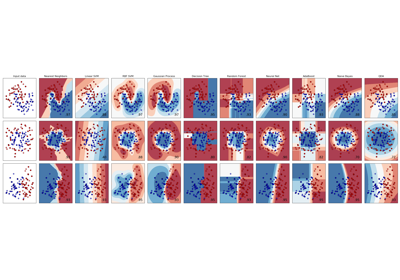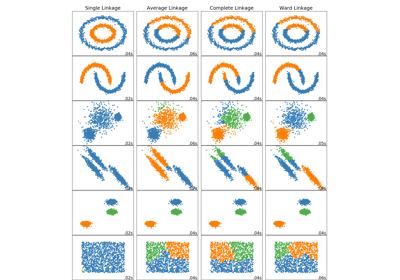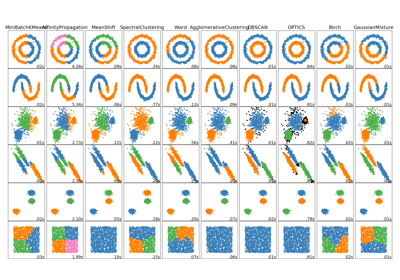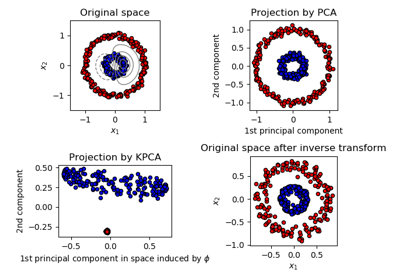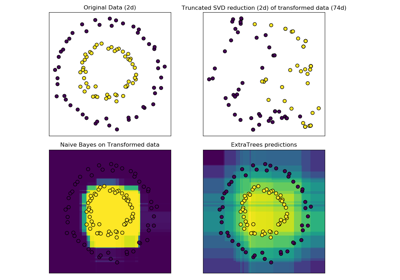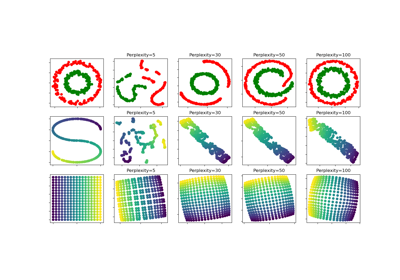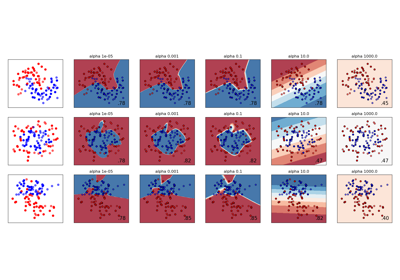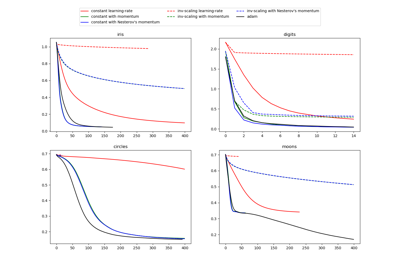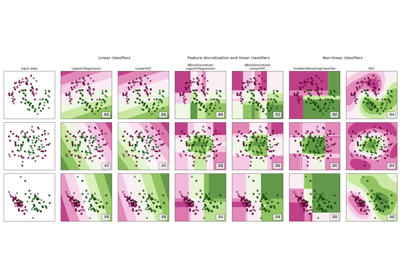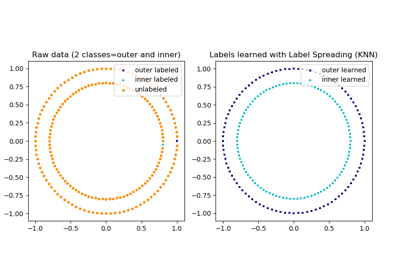# sklearn.datasets.make_circles¶

sklearn.datasets.make_circles(n_samples=100, shuffle=True, noise=None, random_state=None, factor=0.8)[source]

Make a large circle containing a smaller circle in 2d.

A simple toy dataset to visualize clustering and classification algorithms.

Read more in the User Guide.

Parameters
n_samplesint, optional (default=100)

The total number of points generated. If odd, the inner circle will have one point more than the outer circle.

shufflebool, optional (default=True)

Whether to shuffle the samples.

noisedouble or None (default=None)

Standard deviation of Gaussian noise added to the data.

random_stateint, RandomState instance or None (default)

Determines random number generation for dataset shuffling and noise. Pass an int for reproducible output across multiple function calls. See Glossary.

factor0 < double < 1 (default=.8)

Scale factor between inner and outer circle.

Returns
Xarray of shape [n_samples, 2]

The generated samples.

yarray of shape [n_samples]

The integer labels (0 or 1) for class membership of each sample.

## Examples using sklearn.datasets.make_circles¶